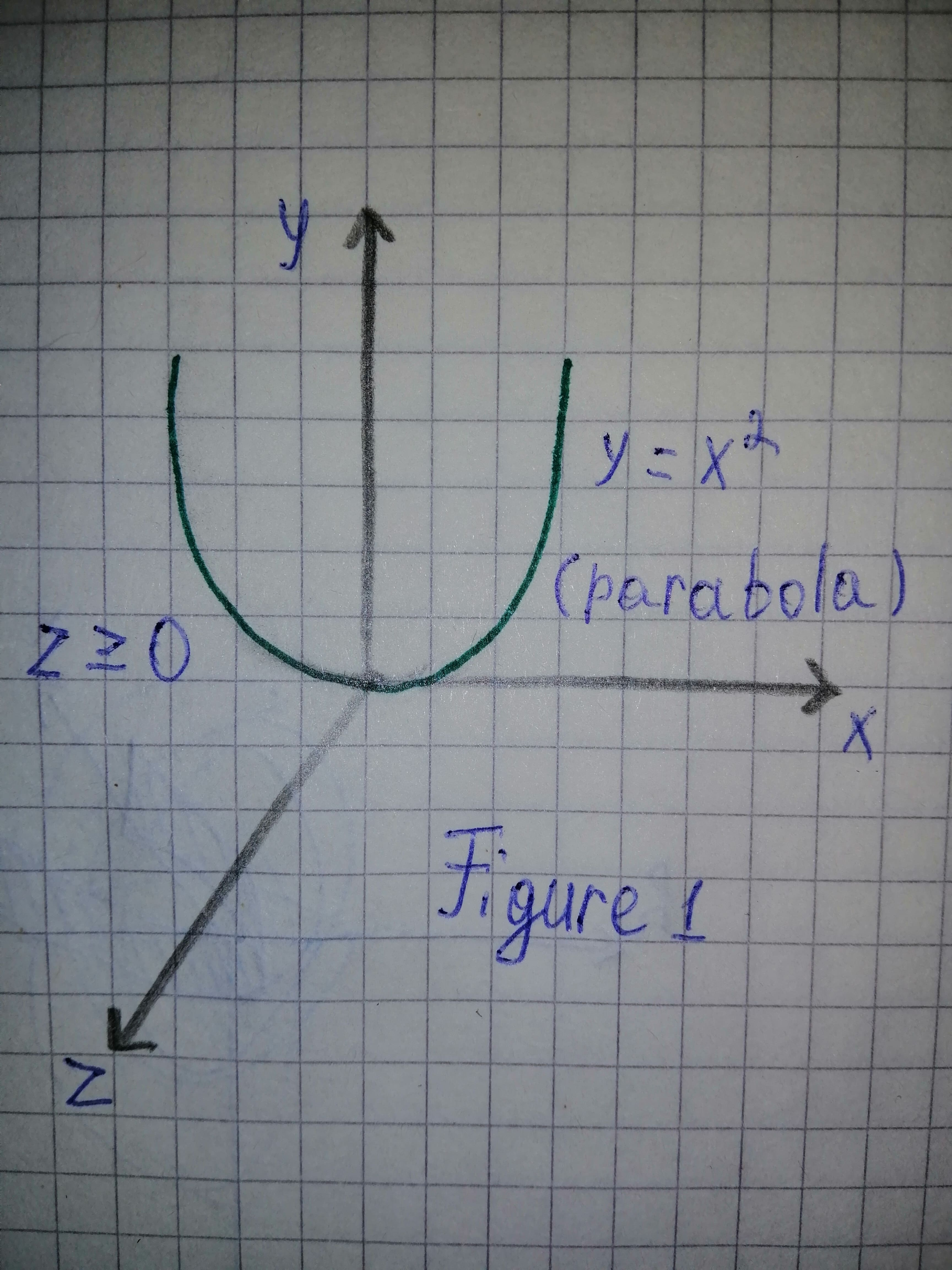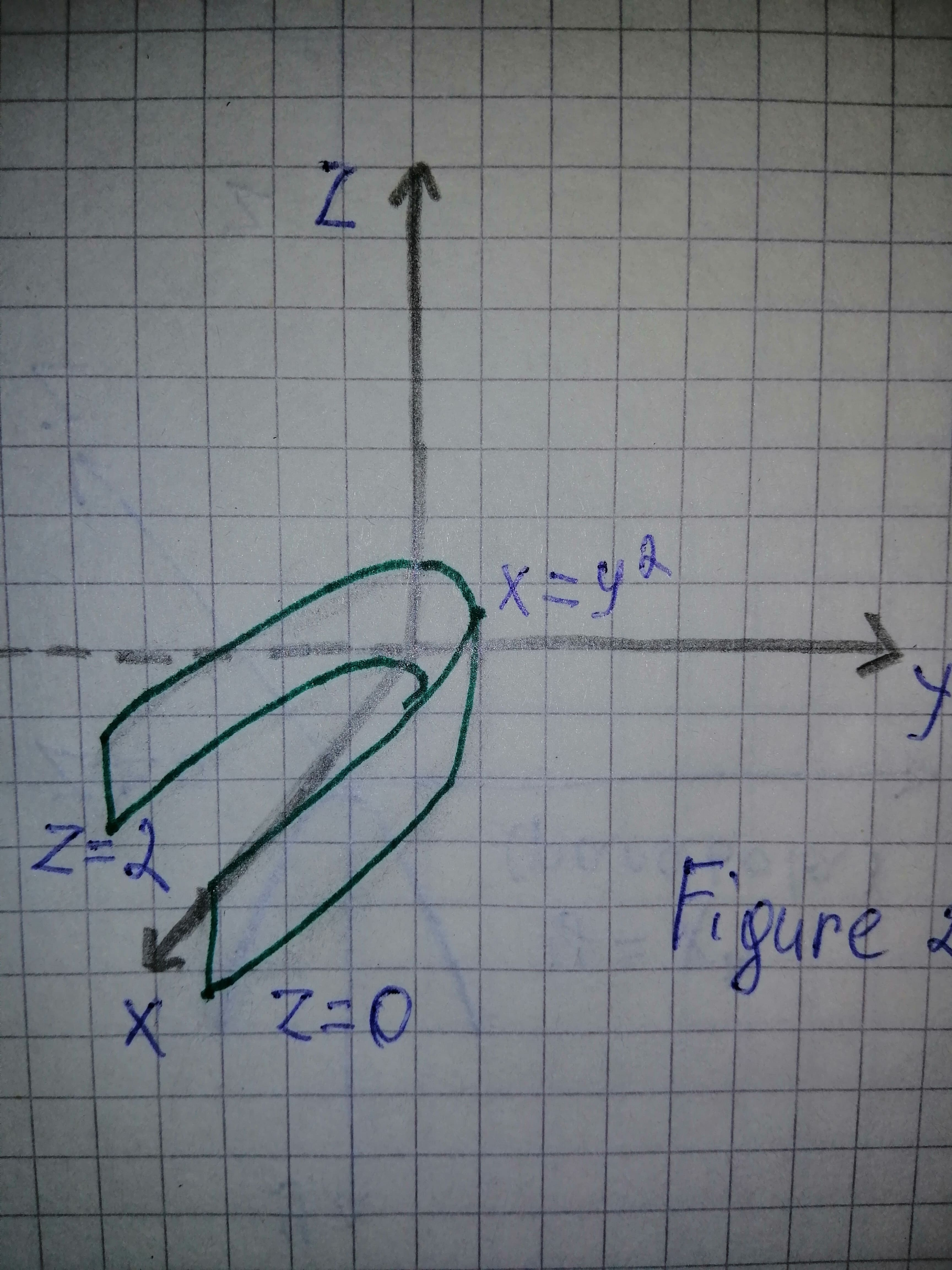# To determine: Find the sets of points in space whose coordinates satisfy the given combinations of equation and inequalities: a) displaystyle{y}ge{x}^{2},{z}ge{0}, b) displaystyle{x}le{y}^{2},{0}le{z}le{2}.Kaycee Roche 2020-11-05 Answered
To determine:
Find the sets of points in space whose coordinates satisfy the given combinations of equation and inequalities:
a) $y\ge {x}^{2},z\ge 0,$
b) $x\le {y}^{2},0\le z\le 2.$
You can still ask an expert for help

• Questions are typically answered in as fast as 30 minutes

Solve your problem for the price of one coffee

• Math expert for every subject
• Pay only if we can solve itAamina Herring

a)
Generally, the plane is determined by the coordinated axis is the xy-plane, whose standard equation by $z=0$.
From equation $y\ge {x}^{2},z\ge 0,y={x}^{2}$ represent the parabola curve in the xy-plane and all point above the region $z=0.$
Figure 1 shows for $y\ge {x}^{2},z\ge 0$ in the Cartesian coordinate system.Thuse, the sets of points in space whose coordinates satisfy the given combinations of equations and inequalities for $y\ge {x}^{2},z\ge 0$ is the region inside the parabola $y\ge {x}^{2}$ in the xy-plane.
b)
From equation $x\le {y}^{2},0\le z\le 2,x={y}^{2}$ represent the parabola curve in the xy-plane in the region on or to the left of the parabola and also have all point above the region from $z=0\to z=2.$
Figure 2 shows for $x\le {y}^{2},0\le z\le 2$ in the Cartesian coordinate system.Thus, the sets of points in space whose coordinates satisfy the given combinations of equations and inequalities for $x\le {y}^{2},0\le x\le 2$ is the region on or to the left the parabola $x\le {y}^{2}$ in the xy-plane and all points from $z=0\to z=2.$